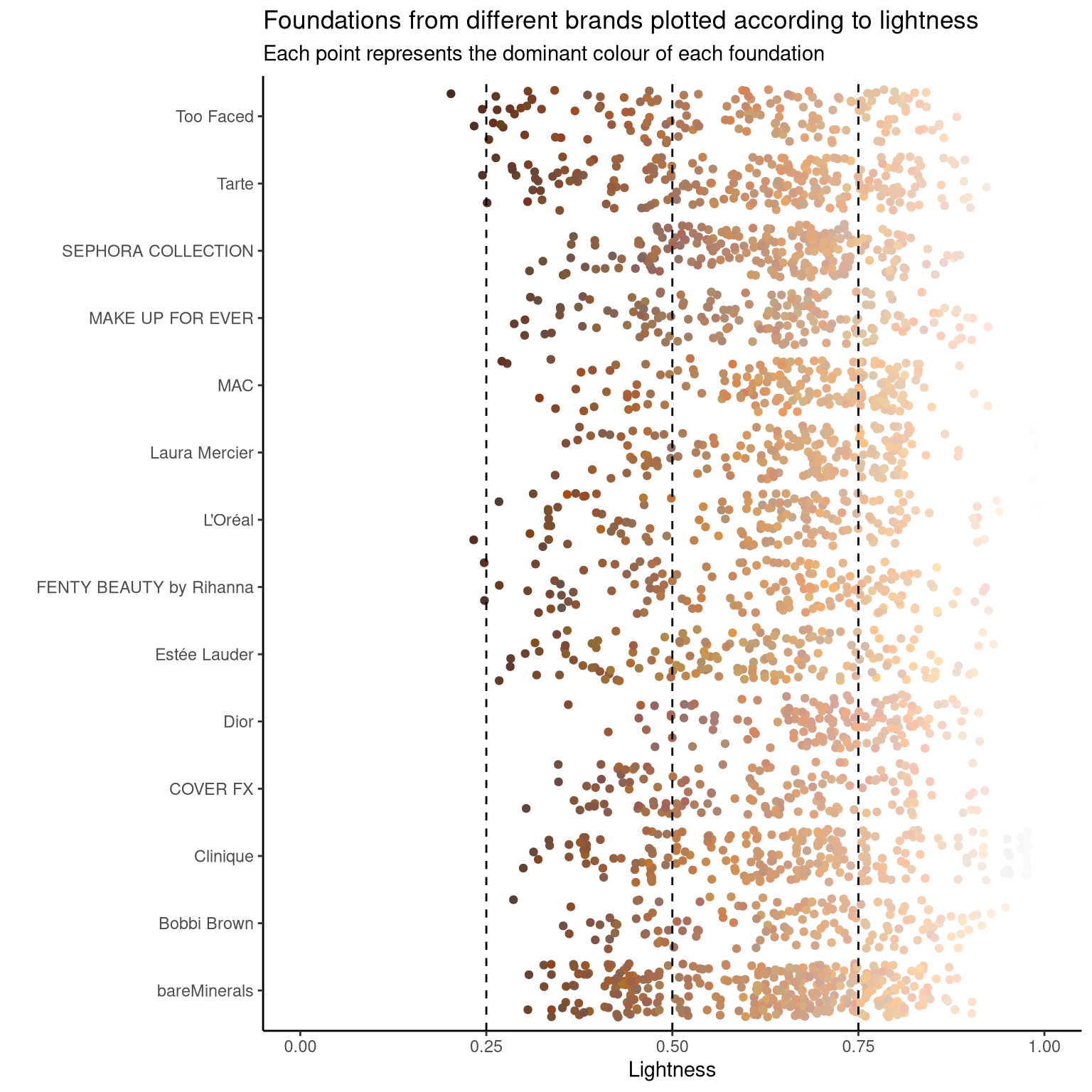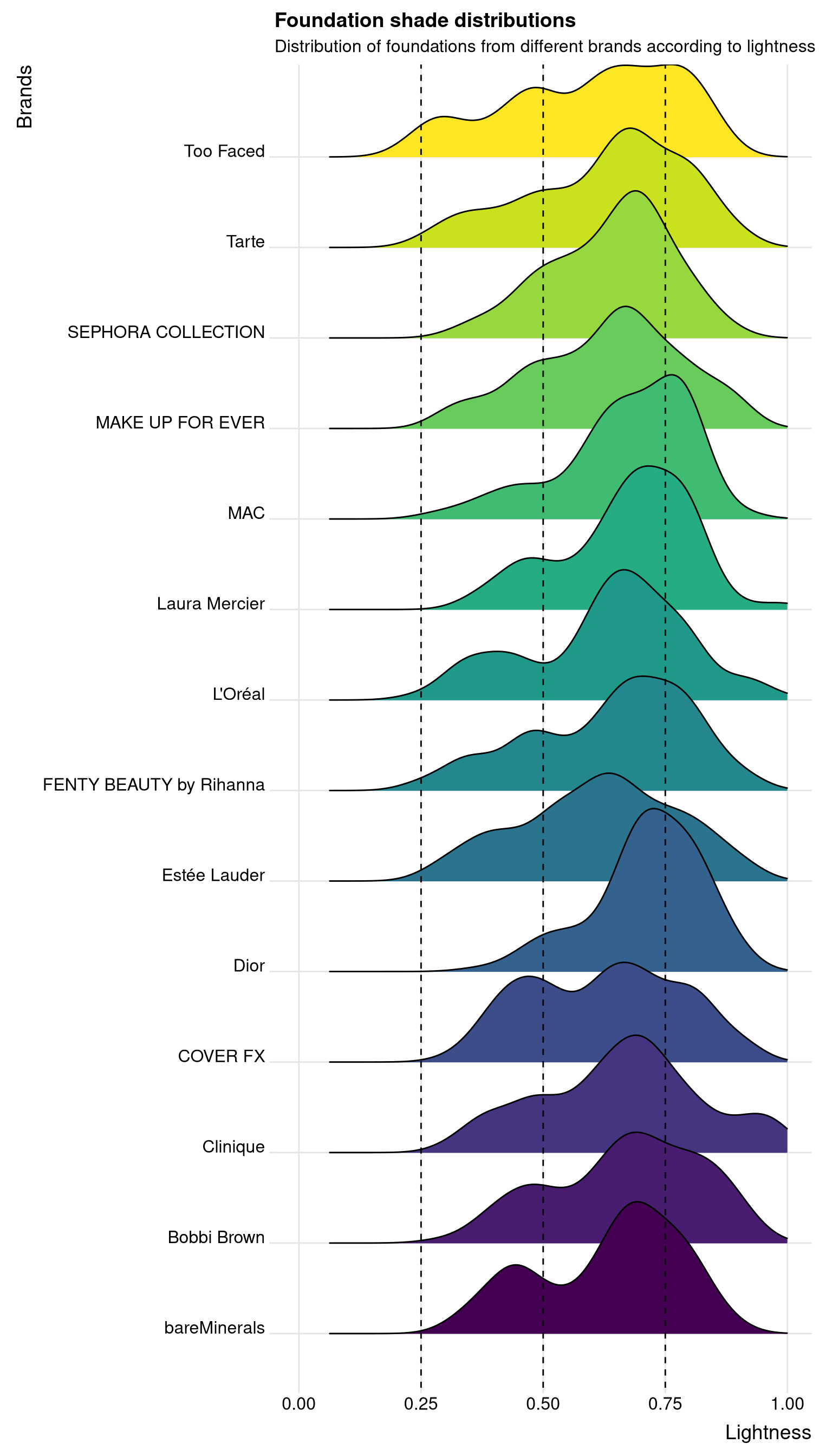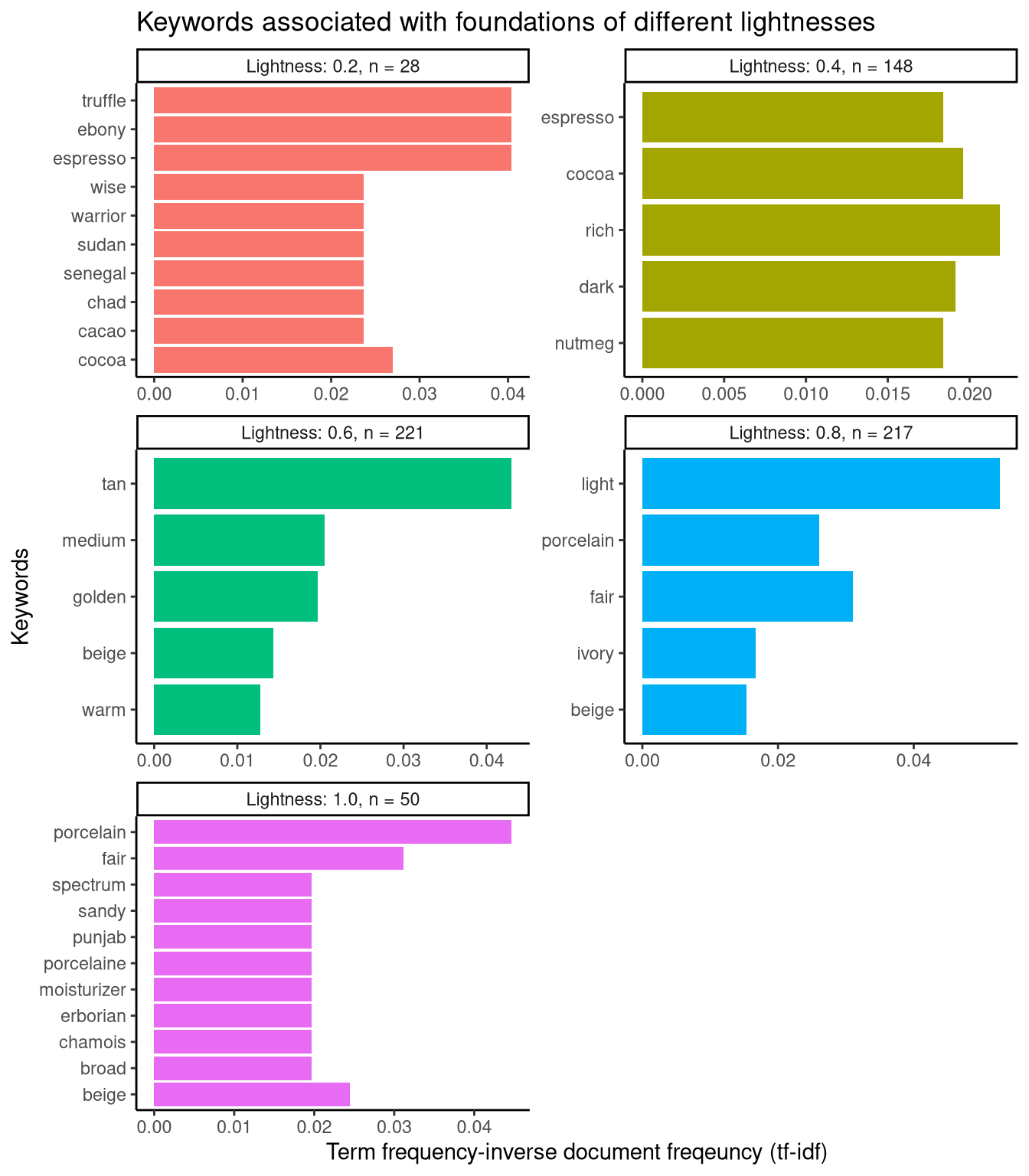``` ```

# Plotting foundations according to shade

Graphs and analysis using the #TidyTuesday data set for week 14 of 2021 (30/3/2021): “Makeup Shades”

Ronan Harrington https://github.com/rnnh/
04-06-2021

## Setup and data preparation

Loading the `R` libraries and data set.

Show code
``````# Loading libraries
library(tidyverse)
library(tidytuesdayR)
library(viridis)
library(tidytext)
library(forcats)
library(ggridges)

``````
``````

Wrangling data for visualisation.

Show code
``````# Selecting the 14 brands with the most foundations in the data set as
# "top_brands"
select(brand) %>%
count(brand) %>%
slice_max(order_by = n, n = 14)

# Selecting foundation names broken into individual words and lightness values
# rounded to the nearest significant digit as "simplified_names"
mutate(rounded = signif(lightness, digits = 1)) %>%
filter(!is.na(name)) %>%
filter(rounded %in% c(0.2, 0.4, 0.6, 0.8, 1.0)) %>%
select(name, rounded) %>%
unnest_tokens(word, name) %>%
count(rounded, word, sort = T)

# Counting the total number of words per rounded lightness value
total_words <- simplified_names %>%
group_by(rounded) %>%
summarise(total = sum(n))

# Added word count totals and tf-idf values to "simplified_names", and changing
# "rounded" to a factor variable with informative levels
simplified_names <- left_join(simplified_names, total_words, by = "rounded")
simplified_names <- simplified_names %>%
bind_tf_idf(word, rounded, n)
simplified_names\$rounded <- as.factor(simplified_names\$rounded)
table(simplified_names\$rounded)
``````
``````
0.2 0.4 0.6 0.8   1
28 148 221 217  50 ``````
Show code
``````levels(simplified_names\$rounded) <- c("Lightness: 0.2, n = 28",
"Lightness: 0.4, n = 148",
"Lightness: 0.6, n = 221",
"Lightness: 0.8, n = 217",
"Lightness: 1.0, n = 50")
simplified_names
``````
``````# A tibble: 664 x 7
rounded                 word       n total     tf   idf tf_idf
<fct>                   <chr>  <int> <int>  <dbl> <dbl>  <dbl>
1 Lightness: 0.8, n = 217 light    156  1512 0.103  0.511 0.0527
2 Lightness: 0.6, n = 221 medium   129  1401 0.0921 0.223 0.0205
3 Lightness: 0.6, n = 221 tan      118  1401 0.0842 0.511 0.0430
4 Lightness: 0.8, n = 217 ivory    113  1512 0.0747 0.223 0.0167
5 Lightness: 0.8, n = 217 beige    104  1512 0.0688 0.223 0.0153
6 Lightness: 0.4, n = 148 deep      99   748 0.132  0     0
7 Lightness: 0.8, n = 217 fair      92  1512 0.0608 0.511 0.0311
8 Lightness: 0.6, n = 221 beige     90  1401 0.0642 0.223 0.0143
9 Lightness: 0.8, n = 217 warm      82  1512 0.0542 0.223 0.0121
10 Lightness: 0.6, n = 221 warm      80  1401 0.0571 0.223 0.0127
# … with 654 more rows``````

## Plotting foundations according to lightness

In this plot, each point represents a single foundation from the 14 most represented brands in the data set. The colour of each point corresponds to the dominant shade of each foundation. These points are arranged according to the lightness of each foundation.

Show code
``````# Plotting all the foundations from "top_brands" according to lightness
filter(brand %in% top_brands\$brand) %>%
ggplot(aes(lightness, brand, colour = hex)) +
geom_jitter() +
scale_colour_identity() +
xlim(0, 1) +
theme_classic() +
geom_vline(xintercept = 0.25, linetype = "dashed") +
geom_vline(xintercept = 0.50, linetype = "dashed") +
geom_vline(xintercept = 0.75, linetype = "dashed") +
labs(y = "", x = "Lightness",
title = "Foundations from different brands plotted according to lightness",
subtitle = "Each point represents the dominant colour of each foundation")
``````## Plotting distributions of foundation lightness

In this plot, the distributions of foundations from the brands in the previous graph are plotted according to lightness. Across all these brands, lighter shades are more represented than darker shades.

Show code
``````# Plotting the distribution of foundations from "top_brands" according to
# lightness
filter(brand %in% top_brands\$brand) %>%
ggplot(aes(lightness, brand, fill = brand, group = brand)) +
scale_fill_viridis(discrete = TRUE) +
xlim(0, 1) +
theme_ridges() +
geom_vline(xintercept = 0.25, linetype = "dashed") +
geom_vline(xintercept = 0.50, linetype = "dashed") +
geom_vline(xintercept = 0.75, linetype = "dashed") +
theme(legend.position = "none") +
labs(y = "Brands", x = "Lightness",
subtitle = "Distribution of foundations from different brands according to lightness")
``````## Plotting keywords associated with foundations of different shades

In this section, keywords associated with foundations of different shades are plotted. This is done by…

• taking all the available foundation names as a corpus
• splitting that corpus into different documents based on rounded lightness values
• calculating tf-idf to find significant words used to describe foundations according to their shade

From this plot, we can see that the darkest (“Lightness: 0.2”) and lightest (“Lightness: 0.8”) foundations are associated with more descriptive, unique keywords than the intermediate shades.

Show code
``````simplified_names %>%
group_by(rounded) %>%
slice_max(n = 5, order_by = tf_idf) %>%
ungroup() %>%
ggplot(aes(tf_idf, fct_reorder(word, tf_idf), fill = rounded)) +
geom_col(show.legend = FALSE) +
facet_wrap(~rounded, ncol = 2, scales = "free") +
theme_classic() +
labs(x = "Term frequency-inverse document freqeuncy (tf-idf)", y = "Keywords",
title = "Keywords associated with foundations of different lightnesses")
``````### Corrections

If you see mistakes or want to suggest changes, please create an issue on the source repository.

### Reuse

Text and figures are licensed under Creative Commons Attribution CC BY 4.0. Source code is available at https://github.com/rnnh/TidyTuesday/, unless otherwise noted. The figures that have been reused from other sources don't fall under this license and can be recognized by a note in their caption: "Figure from ...".

### Citation

`Harrington (2021, April 6). Ronan's #TidyTuesday blog: Plotting foundations according to shade. Retrieved from https://tidytuesday.netlify.app/posts/2021-04-06-makeup-shades/`
```@misc{harrington2021plotting,